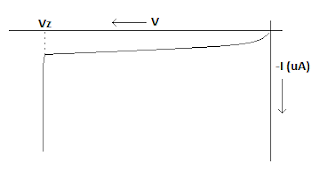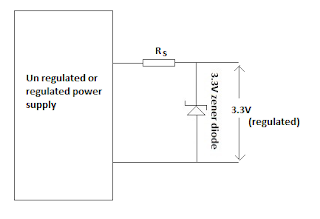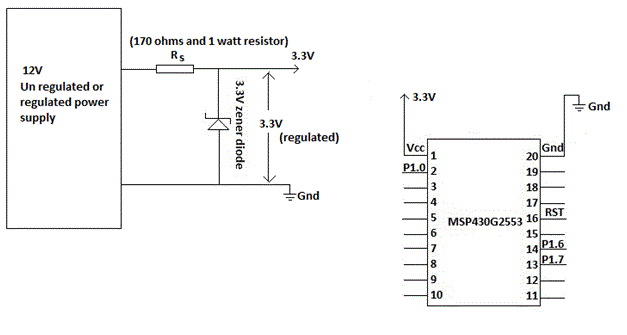### Simple way to achieve 3.3V regulated DC power supply for MSP430 Microcontrollers

Simple way to achieve 3.3V regulated DC power supply for MSP430 Microcontrollers
Introduction:
The operating voltage for the MSP430 Microcontrollers is range from 1.8V to 3.6V DC supply. The Frequency of operation requires maximum voltage range (3.6V).

The above figure shows that the Clock frequency should be matched with the required supply voltage, otherwise the unreliable execution will results.  Off course Low Drop-Out (LDO) voltage regulators are required for MSP430 to achieve low power. The LDO’s are the voltage regulators in which drop out voltage is very less. Generally, regular 7812 (12v regulator), 7805 and others are not comes under LDO’s because these regulators require high input voltage than output. The 7812 regulator if self require the input voltage of 14v, therefore the drop out voltage is 2V to achieve 12V regulated DC output.
Example for a 3.3 LDO regulator is LM3111.

Anyway here I am not developing a low drop out regulator, but here I am just prepared the 3.3 V regulated power supply using shunt zener diode. Even though it was not efficient when compare to LDO, but this circuit was simple and readily prepared.

Generally, we do find a lot if DC power supply regulators in the market like 7812 (12 volts regulator), 7805 (5V regulator), LM3111 (3.3V LDO regulator) , etc. But sometimes we don’t readily find the regulators in your house or nearby electronics shops.

Then what we will do?
Don’t worry here we have the solution by using the zener shunt voltage regulator – easily made regulator.

Simple Introduction to Zener Diode:
A zener diode is not regular diode because it allows the current in the reverse biased operation. If the reverse bias voltage reaches the break down voltage (Vz) then the huge amount of current (Izmax) starts to flow through the zener diode. Even if we increase the reverse voltage the voltage across the zener is constant but the current will increase very drastically as shown in the Fig 1.2. Hence the zener diode is referred as constant-voltage source. A zener diode is specified by its maximum power dissipation and its break down voltage.Figure 1.2 Zener diode reverse biased characteristics

Zener Voltage regulator Circuit Diagram:Figure 1.3 zener diode voltage regulator circuit

Circuit Explanation:
In the above figure 1.3 a series resistance is connected to the un regulator power supply to limit the current flowing in the zener diode which is connected in shunt. In the figure 1.3, I have shown you that un-regulated or regulated power supply box (left side. That is just for you that you can use either of the supply to obtain 3.3V voltage.

Why power supply regulation is required?
If the output voltage in power supply is not regulated, the voltage will reduces as the load current increases. Here four important points should be remembered to design this circuit.
1. How much output voltage is required to drive your Microcontroller?
This question will answer the voltage of zener diode to be used. MSP430 microcontroller will requires a voltage range 1.8 to 3.6 V, therefore a 3.6V or 3.3V zener diode is chosen.
2. How much current you need to drive your microcontroller and its connected peripherals?
This question will answer the value of series resistance (Rs) that should be connected.  Let us suppose, if your Microcontroller is going to drive a 16x2 character LCD, assume that it require 50 to 100mA. Then the resistor value is given by Rs = (X – Vz)/ILoad.
X  - voltage of the unregulated supply,
Vz  - zener diode break down voltage = 3.3V,
ILoad – load current (example : LED, LCD) = 50mA for LCD
Rs – series resistance(?) – To be calculated.
If X is taken as 5V regulated or un regulated supply, then
Rs = (5V-3.3V)/50mA = 34 ohms.
If X is taken as 12V regulated or un-regulated supply, then
Rs = (12V-3.3V)/50mA = 174 ohms.

3. How much power dissipated by the series resistor (Rs)?
This question will answer the wattage of the resistor. The power dissipation in the resistor is calculated by the given formulae.
For a 5 volt un-regulated power supply
Presistor = Vresistor  X ILoad;
= (5 - 3.3) x 50mA = 0.085 watt
For a 12 volt un-regulated power supply
Presistor = Vresistor X ILoad;
= ( 12 - 3.3) x 50mA = 0.435 watt
Therefore the resistor should be half watt resistor or greater is required to use in this circuit.

4. What about the zener diode wattage?
Here, the zener diode voltage is 3.3V and the current flowing through it 50mA.
Therefore the power dissipation in the zener diode is given by
Pzener = Vz x ILoad = 3.3 x 30mAFigure 1.4 a 3.3V power supply for the MSP430 MCU.

#### 1 comment :

1.I have bookmarked your blog

Thanks for visiting NPEDUCATIONS. We will contact you soon

#### 1 comments :

Herbert Arnold said...

I have bookmarked your blog